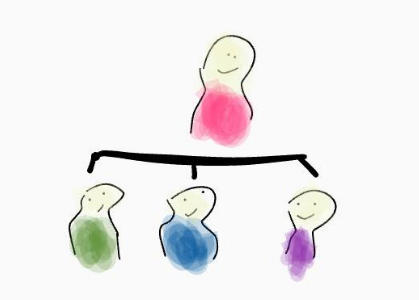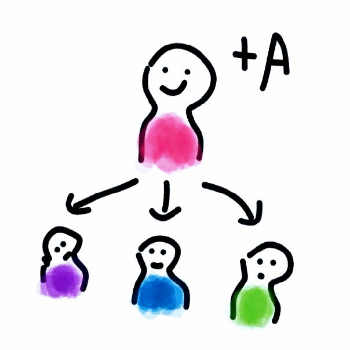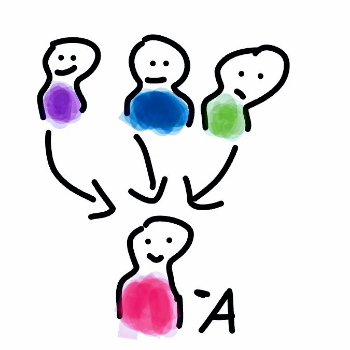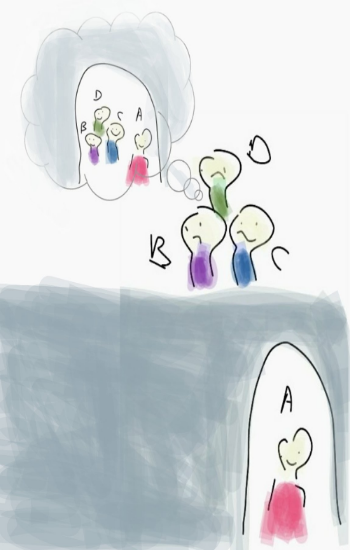Connecting...

# Variances in Scala by Wiem Zine El Abidine

There is a lot to learn within the Scala programming language and many things it supports. Software Engineer Wiem Zine El Abidine breaks down a few of the variances in Scala including Types, Subtypes, Function type and more.

Variance refers to how subtyping between more complex types relates to subtyping between their components” — Wikipedia

Types

A value is the result of an expression, a type is a set of values. Each value in our program has a type, and every type has a finite/infinite possible values. For instance:

• Boolean is a type that has two possible values: 'true' or 'false'
• String has infinite possible values.
• This sum type is a set of 3 values, '[Circle, Triangle, Rectangle]'
``````sealed trait Shape
case object Circle    extends Shape
case object Triangle  extends Shape
case object Rectangle extends Shape``````
• Product type: 'Container' has a combination of n values of type 'Shape' with m values of type 'Boolean' → 3 * 2 = 6 possible values.
``case class Container(form: Shape, hasBg: Boolean)``

Subtypes

Scala supports subtyping.

In our programming daily life, we encounter subtypes like:

• 'Some' and 'None' are subtypes of 'Option'
• In the previous example, 'Circle, Triangle' and 'Rectangle' are subtypes of 'Shape'
• 'Nothing' is the subtype of every type and there is no instances of this type.
• 'Any' is the supertype of every type in Scala. It’s the root of the Scala class hierarchy. So every type is a subtype of 'Any'.More complex types

A simple type:

``case class Person(id: Int, name: String)``

'Person' is called a type constructor with 0 arity.

Higher kinded type (HKT)

It’s about how to classify the type constructors:

• 'Person' is kind (star) '*' the type constructor has no type parameters
• 'Option[A]' is kind (star to star): '* => *' because 'Option' has one parameter, like the function 'f(a: A): B' is 'f: A => B'
• 'Map[K, V]' is kind (star star to star)'[*, *] => *' , like the function 'f(k: K, v: V): R' is 'f: (K, V) => R'
PS: I learned those and more in the day 1 of Functional Scala training by John De Goes.

Variance annotation

Depending to the variance of the type constructor, the subtyping relation can be either preserved (covariance), reversed (contravariance), or ignored (invariance).

CovarianceContravarianceInvarianceFunction type

Let’s define a simple function:

``````scala> val addOne = (i: Int) => i + 1
addOne: Int => Int = <function1>``````

First class functions in Scala are implemented as 'FunctionX' classes. ( 'X' equals to the number of its argument)

In this example, we have a function with one parameter, the compiler picks 'Function1[Int, Int]' as the underlying typer.

'Function1' has this type signature: 'Function[-A, +B]'

'-' and '+' are variance annotations

'Function[-A, +B]' is:

• Contravariant in the input type 'A' (marked with '-') where 'A' can be replaced with the derived type. (more general input argument)
• Covariant in the output type 'B' (marked with '+' ) where 'B' can be replaced with the base type. (more specific return type)

Following this rule:

“Be conservative in what you do, be liberal in what you accept from others” — robustness principle

Upper and Lower type bounds

Upper type bounds: 'A <: T' means that 'A' refers to a subtype of 'T'

Example:

``````abstract class Pet extends Animal {}
case class Cat(???) extends Pet
case class Dog(???) extends Pet

trait Zoo[T <: Pet]{
...
}``````

Lower type bounds: 'A >: T' means that 'A' refers to a supertype of 'T'

We will see an example soon.

In order to understand the previous sections, we need to see a simple example.

Let’s re-implement the type 'List'.

``````abstract class List[T] { ... }
case class Nil[T]() extends List[T]
case class Cons[T](head: T, tail: List[T]) extends List[T]``````

The type parameter at 'List' is not marked covariant or contravariant. So 'List' is invariant in 'T' which means List can only have elements of that type = 'T' cannot be changed.

``````sealed trait A
case object B extends A
case object C extends A
case object D extends A``````

If we want to define a list of type A that has elements of its subtypes we’ll get a compile error.

``val list: List[A] = Cons(B, Cons(C, Cons(D, Nil()))) //compile error: class List is invariant in type A.``To make their dream come true, 'List' of the subtypes of 'A' should be represented as a 'List[A]'

In simple types, we’re able to define: 'val a: A = B'

But in HKT we have to add the variance annotation '+' to the type parameter of our generic class 'List' to make it covariant in its type 'T': 'List[+T]'

``````abstract class List[+T] { ... }
case class Nil[T]() extends List[T]
case class Cons[+T](head: T, tail: List[T]) extends List[T]``````

Before checking if it works, let’s improve the 'case class Nil()'

Every type T is a supertype of Nothing . 'List' is covariant in 'T', so we can say that a 'List[Nothing]' is a subtype of 'List[T]'.

'Nil' doesn’t use the type parameter, it could 'extends List[Nothing]'

``case object Nil extends List[Nothing] ``

Looks nicer!

``````val list: List[A] = Cons(B, Cons(C, Cons(D, Nil)))) //it works :)
``````

Now let’s implement a function 'contains' in 'List' that checks if a given element exists.

``````abstract class List[+T] { self =>
def contains(elem: T): Boolean = self match {
case Cons(x, xs) if x == elem => true
case Cons(x, xs) => xs.contains(elem)
case Nil         => false
}
}``````

Oups there is a compile error :

``````error: covariant type T occurs in contravariant position in type T of value elem
def contains(elem: T): Boolean = self match {``````

I talked previously about 'Function[-A, +B]' and mentioned that the inputs of functions are contravariant and their output are covariant. In our case, 'List' is covariant in 'T',

The problem is in the input argument of 'contains' the element is a value of type 'T' which is covariant, we need to make it contravariant to be able to pass it into 'Function1'

We can do that using lower type bounds '>:' !

``````abstract class List[+T] { self =>
def contains[T1 >: T](elem: T1): Boolean = self match {
case Cons(x, xs) if x == elem => true
case Cons(x, xs) => xs.contains(elem)
case Nil         => false
}
}``````

it works!

If you have a contravariant type parameter and you need to define a function that returns a value of that type, you’ll need to use the term '<:' to make the output covariant.

I hope that you enjoyed reading this blog and now you’ll not worry when you see '[+T],[-T], <:, >:' in code.This article was written by Wiem Zine El Abidine and posted originally on Medium